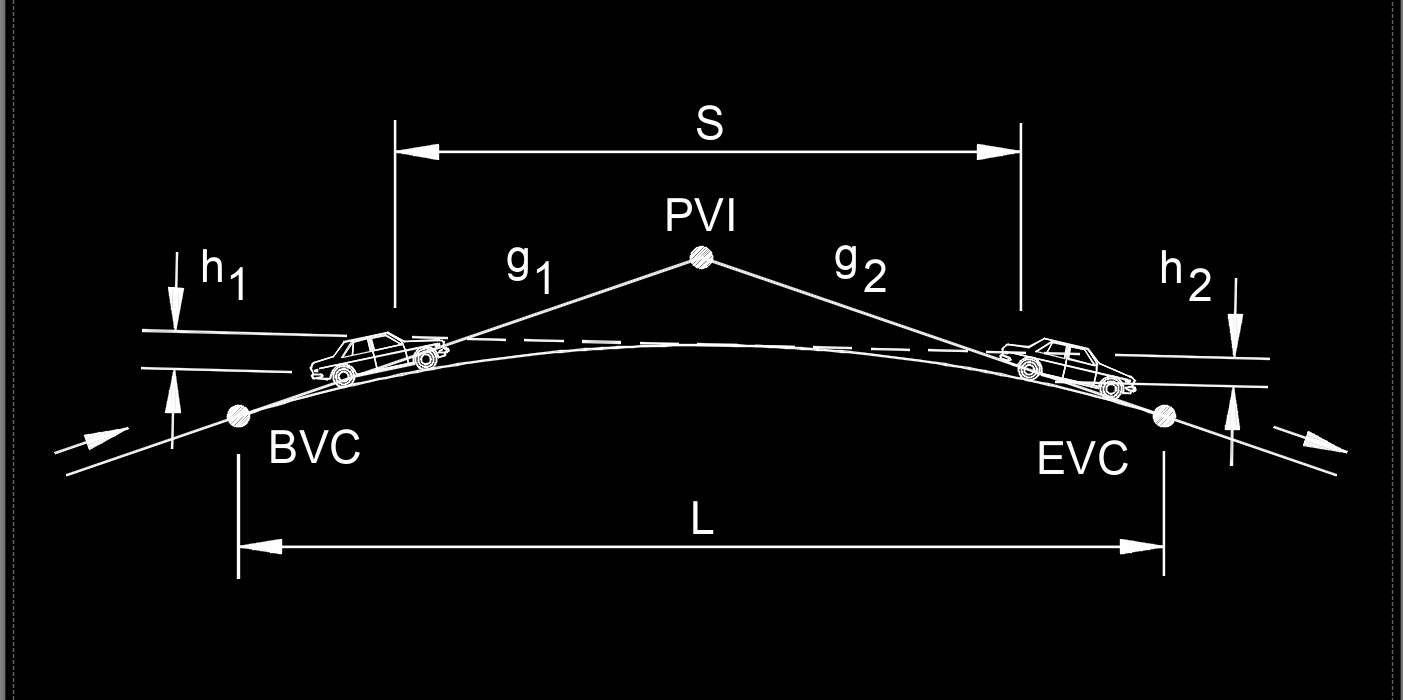# K Value

on . Posted in Civil EngineeringK value, abbreviated as K, is the horizontal distance required to achieve a 1% change in the slope of the vertical curve.

## K Value formula

$$\large{ K = \frac{ L }{ A } }$$
Symbol English Metric
$$\large{ K }$$ = rate of vertical curvature  $$\large{ft}$$  $$\large{m}$$
$$\large{ L }$$ = length of vertical curve $$\large{ft}$$ $$\large{m}$$
$$\large{ A }$$ = algebric difference in grade (%) $$\large{dimensionless}$$